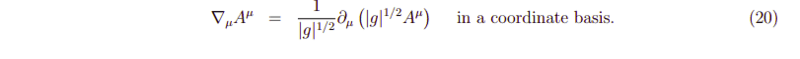# Covariant derivative in coordinate basis

LCSphysicist
Homework Statement:
.
Relevant Equations:
.I need to evaluate ##\nabla_{\mu} A^{\mu}## at coordinate basis. Indeed, i should prove that ##\nabla_{\mu} A^{\mu} = \frac{1}{\sqrt(|g|)}\partial_{\mu}(|g|^{1/2} A^{\mu})##.

So, $$\nabla_{\mu} A^{\mu} = \partial_{\mu} A^{\mu} + A^{\beta} \Gamma^{\mu}_{\beta \mu}$$

The first and third terms of Christoffel will cancel, so $$= \partial_{\mu}A^{\mu} + A^{\beta} \frac{g^{\mu x}}{2}(\partial_{\beta}g_{x \mu})$$

Now, using the fact that ##\delta g = g g^{\mu v} \delta g_{\mu v}##, we can easily find that $$\frac{g^{\mu x}}{2}(\partial_{\beta}g_{x \mu}) = \frac{\partial_{\beta}(|g|^{1/2})}{|g|^{1/2}}$$

After substitute this at our main expression, we can recover ##\nabla_{\mu} A^{\mu} = \frac{1}{\sqrt(|g|)}\partial_{\mu}(|g|^{1/2} A^{\mu})##.

The problem is, i have no idea what assumption i have made so that my result applies only to coordinate basis! That is, the problem ask for prove it at coordinate basis , so i guess it should be true only at these type of basis. But i haven't assumed nothing, just manipulate the terms and got the result. What am i missing? Is this expression really general like i have found?

Staff Emeritus
Homework Helper
Gold Member
You have used the coordinate expression for the Christoffel symbols.

•LCSphysicist
LCSphysicist
You have used the coordinate expression for the Christoffel symbols.
I know there are another expressions for Christoffel symbol, like:
$$\Gamma^{i}_{jk} = - \partial e^{i}/\partial x^{j} e_{k}$$
But i didn't know that this expression i have used is not the most general. What is the most general expression for it so? (namely, the one that makes no reference to any frame)